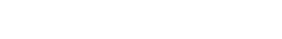# Maths MCQs for Class 12 with Answers Chapter 5 Continuity and Differentiability

Free PDF Download of CBSE Maths Multiple Choice Questions for Class 12 with Answers Chapter 5 Continuity and Differentiability. Maths MCQs for Class 12 Chapter Wise with Answers PDF Download was Prepared Based on Latest Exam Pattern. Students can solve NCERT Class 12 Maths Continuity and Differentiability MCQs Pdf with Answers to know their preparation level.

## Continuity and Differentiability Class 12 Maths MCQs Pdf

1. Given functions f(x) = $$\frac{x^{2}-4}{x-2}$$ and g(x) = x + 2, x <= R. Then which of the following is correct?
(a) f is continuous at x = 2,
g is continuous at x = 2
(b) f is continuous at x = 2,
g is not continuous at x = 2
(c) f is not continuous at x = 2,
g is continuous at x = 2
(d) f is not continuous at x = 2,
g is not continuous at x = 2

Explaination: (c), as f(2) is not defined so / is not continuous at x = 2 ‘g’ is a polynomial function, so continuous at x = 2.

2.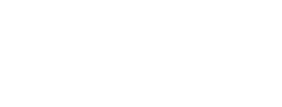Explaination: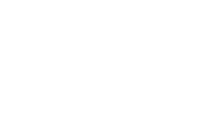3.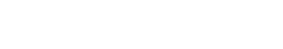for x = 2, then value of k for which f is continuous is
(a) -2
(b) -1
(c) 0
(d) 1

Explaination: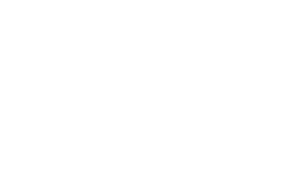4. A function /is said to be continuous for x ∈ R, if
(a) it is continuous at x = 0
(b) differentiable at x = 0
(c) continuous at two points
(d) differentiable for x ∈ R

Explaination: (d), as differentiable functions is continuous also.

5. Afunction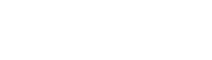is continuous at x = 0 for
(a) k = 1
(b) k = 2
(c) k = $$\frac{1}{2}$$
(d) k = $$\frac{3}{2}$$

Explaination: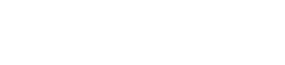6. Write the number of points where f(x) = |x + 2| + |x – 3| is not differentiable.
(a) 2
(b) 3
(c) 0
(d) 1

Explaination: (a), as f(x) = |x – a| is continuous at x = a but not differentiable thereat.

7. Derivative of cot x° with respect to x is
(a) cosec x°
(b) cosec x° cot x°
(c) -1° cosec2 x°
(d) -1° cosec x° cot x°

Explaination: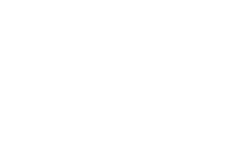8.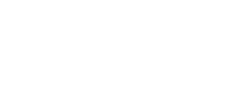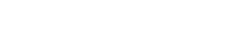Explaination: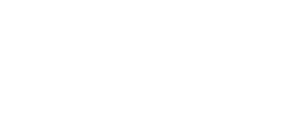9. If f(x) = $$\log _{x^{2}}(\log x)$$, then f(e) is
(a) 0
(b) 1
(c) $$\frac{1}{e}$$
(d) $$\frac{1}{2e}$$

Explaination: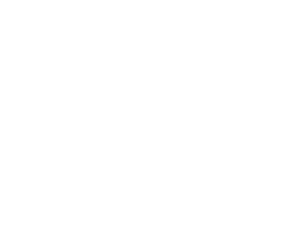10. If f(x) = ex and g(x) = loge x, then (gof)’ (x) is
(a) 0
(b) 1
(c) e
(d) 1 + e

Explaination: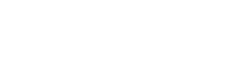11.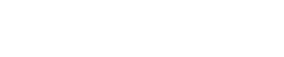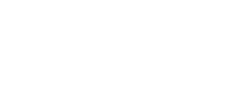Explaination: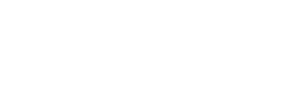12. If y = xx-∞, , then x(l -y log x)$$\frac{d y}{d x}$$ is equal to
(a) x²
(b) y²
(c) xy²
(d) x²y

Explaination: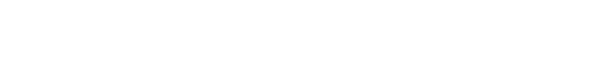13. The derivative of sin x with respect to log x is
(a) cos x
(b) x cos x
(c) $$\frac{\cos x}{\log x}$$
(d) $$\frac{1}{x}$$ cos x

Explaination: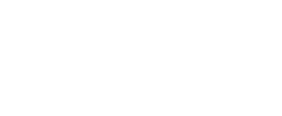14. Ify = Ae5x,+ Be-5x x then $$\frac{d^{2} y}{d x^{2}}$$ is equal to
(a) 25y
(b) 5y
(c) -25y
(d) 10y

Explaination: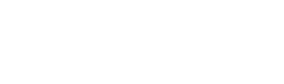15. Given the function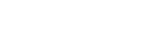the function is continuous at x = 0, state true or false.

Explaination: False, as ‘f’ is not defined at x = 0. i.e.f (0) does not exist.

46. The function f(x) = $$\frac{x+1}{1+\sqrt{1+x}}$$ continuous at x = 0 if/(0) is _________ .

Explaination: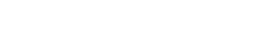17. A function f(x) = $$\frac{x}{x-5}$$ x ∈ R, is a continuous function. State true or false.

Explaination: False, as for x = 5, f(5) is not defined.

18. A function f(x) = sin x + cos x is continuous function. State true or false.

Explaination: True, as sum of two continuous functions is a continuous function.

19. Discuss the continuity of the function fix)= $$\frac{1}{x-5}$$ for x ∈ R.

Explaination: f(x) = $$\frac{1}{x-5}$$, as f(5) is not defined, therefore function is not continuous at x = 5.

20. Discuss the continuity of the function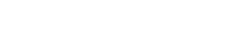Explaination:
f(x)= $$\frac{x^{2}-25}{x-5}$$, x ≠ 5. As x ≠ 5, therefore, value of function exists for all x(≠5) ∈ R.
Also $$\lim _{x \rightarrow a}$$ f(x) = f(a) = a + 5, (a ≠ 5). Hence, continuous.

21. Check whether the function f(x) = 2x² + 1 is continuous at x = 0.

Explaination: f(x) = 2x² + 1, as $$\frac{x^{2}-25}{x-5}$$ f(x) = f(0) = 1. Hence, continuous

22. Give an example of a function which is continuous but not differentiable at exactly two points.

Explaination:
We know function f(x)=|x – a| is continuous at x = a but not differentiable at x = a.
∴ functions |x| and |x – 1| are continuous but not differentiable at x = 0 and 1.
∴ function is h(x) = |x| + |x – 1|.

23. Determine the value of the constant ‘k’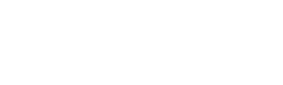Explaination: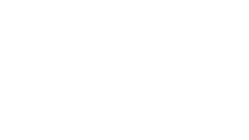24. Determine the value of ‘k’ for which the following function is continuous at x = 3: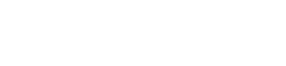Explaination: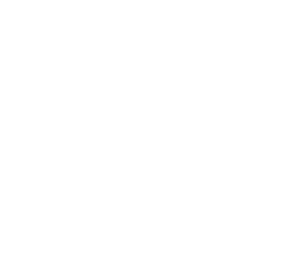25. For what value of ‘k’ is the function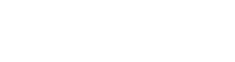continuous at x = 0?

Explaination: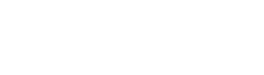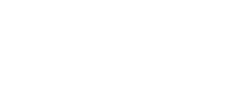26. Find the value of k, so that the function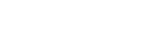is continuous at x = 1

Explaination: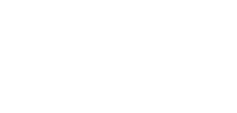27. Determine the value of the constant ‘k’ so that the function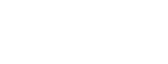is continuous at x = 0. [Delhi]

Explaination: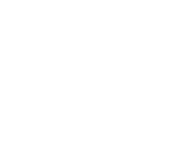28. For what value of ‘k’ is the function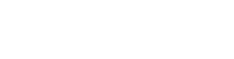continuous at x = 0? [Foreign]

Explaination: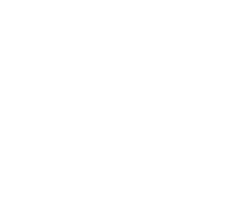29. The derivative of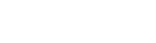State true or false.

Explaination:30. Find $$\frac{d y}{d x}$$, if x² + y² = 5

Explaination: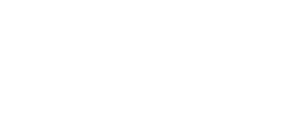31. Differentiate sin-1x², with resepct to x.

Explaination: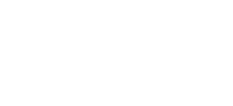32. Find $$\frac{d y}{d x}$$, if sin y + x = log x

Explaination: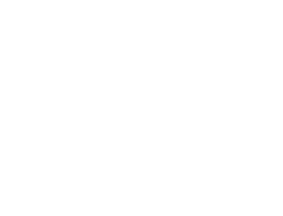33. Find $$\frac{d y}{d x}$$ at x = 1, y = $$\frac{\pi}{4}$$ if sin²y + cos xy = K. [Delhi 2017]

Explaination: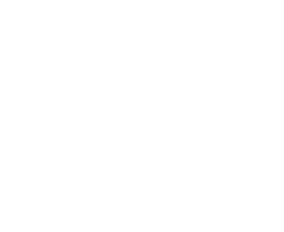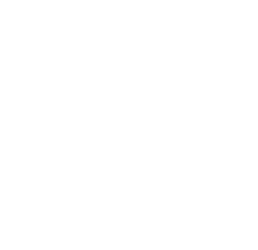34. Differentiate tan-1 $$\left(\frac{1+\cos x}{\sin x}\right)$$ with respect to x. [CBSE 2018]

Explaination: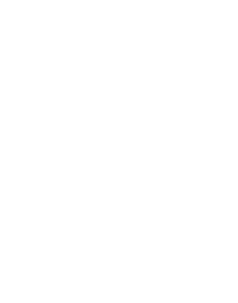35. If y = 2√x, then $$\frac{d y}{d x}$$ is _______ .

Explaination: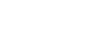36. If y = log (tan x) + log (cot x), then $$\frac{d y}{d x}$$ is _______ .

Explaination: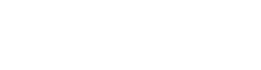37. If $$f(x)=9^{x^{2}+2 x}$$, then f(-1) is _______ .

Explaination: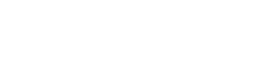38. Differentiate e-2x with respect to x.

Explaination: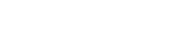39. Differentiate 5sin x, with respect to x.

Explaination: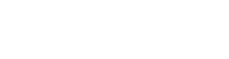40. Differentiate loge(sin x) with respect to x.

Explaination: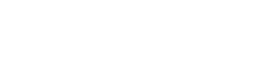41. Differentiate log x² w.r.t x.

Explaination: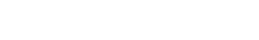42. If y = e-3 log x then find $$\frac{d y}{d x}$$.

Explaination: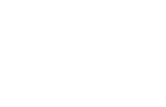43.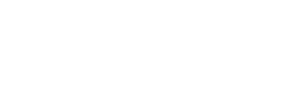Explaination: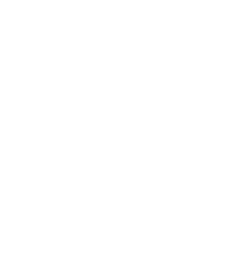44. Find $$\frac{d y}{d x}$$ at t = $$\frac{2 \pi}{3}$$ when x = 10(t – sin t) and y = 12(1 – cos t). [Foreign 2017]

Explaination: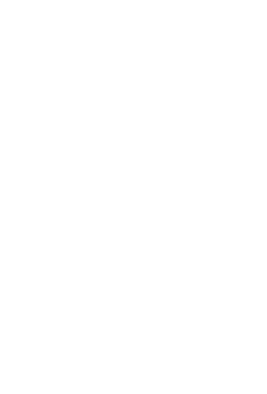45. Find $$\frac{d^{2} y}{d x^{2}}$$, if y = log x

Explaination: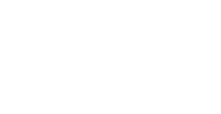46. If y = sin 3x, find y2

Explaination:
y = sin 3x
y1 = 3 cos 3x
y2 = -9 sin 3x.

47. Find $$\frac{d^{2} y}{d x^{2}}$$ if y = e-3x

Explaination: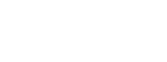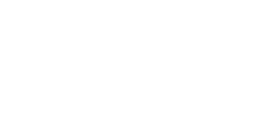48. Verify the Rolle’s Theorem for the function f(x) = x² in the inverval [-1, 1].

Explaination:
Function f(x) = x² is continuous in [-1,1 ], differentiable in ( -1, 1) and f(-1) = f(1). Hence, Rolle’s Theorem verified.
⇒ f'(c) = 0
⇒ 2c = 0
⇒ c = 0 for c ∈ (-1, 1)

49. Verify the Rolle’s Theorem for die functiony(x) = |x| in the inverval [-1, 1]. [HOTS]

Explaination: Not verified, as /(x) =|x| is not derivable at x = 0.

50. Verify the Rolle’s Theorem for the function f(x) = sin 2x in [0, π].